Next: 6.1.2 Organic spin Peierls Up: 6.1 Introduction Previous: 6.1 Introduction

### 6.1.1 Theories

The spin-Peierls transition, which was proposed for an antiferromagnetic S=1/2 spin chain [14,15,16], is characterized by two features: (1) alternating deformation of the lattice along the chain direction, and (2) singlet pair formations of the two S=1/2 spins on the shortened link (Fig.49b). Although the lattice dimerization (1) increases the elastic energy, the transition may still be possible if the singlet pair formation (2) compensates the energy increase. In order to estimate the conditions for spin Peierls transition, a quantum mechanical treatment of the spin system is necessary, because singlet-pair formations are involved in the transition mechanism.A Hamiltonian of a one-dimensional spin chain which allows lattice deformation can be expressed as:

whereis the antiferromagnetic interaction between the spins on the i-th and the-th site, K is the spring constant of the lattice, and ui is the displacement of the lattice point i. The first term of this Hamiltonian describes the spin system and the second term, the elastic energy of the lattice system. Under a lattice deformation along the chain, the antiferromagnetic couplingis expanded as

where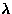is a spin-lattice coupling constant and a is the lattice spacing. The last equation holds for the lattice dimerized state (displacement u) with. Using eq.48 the Hamiltonian (eq.47) is rewritten as:

where, the second term gives a spin-lattice coupling.

Analytical approaches to the spin Peierls problem have been unsuccessful; one popular way to solve the problem is as follows:

(1)
map the spin Hamiltonian to a fermion system using the Jordan-Wigner transformation 
(2)
solve the fermion system, using various approximation techniques developed for fermion systems
(3)
obtain the phonon features, with perturbation treatments of the fermion-phonon coupling, which arose from the spin-lattice coupling (the second term of eq.49).
Since step (2) involves the quantum mechanics of the spin system, this part is the most crucial to this theoretical approach; indeed, the spin-Peierls transition temperature strongly depends on this part.

Bulaevskii  and Pytte  adopted the Hartree approximation for step (2) and calculated the phonon frequency () above the spin Peierls transition temperature. Their result is:

where,is the bare phonon frequency,is a dimensionless spin-lattice coupling parameter and the constants areand. A softening of phonons is predicted, as the result of fermion-phonon coupling. From the temperature which gives a zero phonon frequency, the spin Peierls transition temperature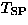has been estimated as:

Since the Hartree approximation drops the exchange interaction between two fermions, it may not adequately include quantum fluctuations. In fact, a quantum mechanical treatment introduced below gives an enhanced spin Peierls transition temperature.

Cross and Fisher  approximated the mapped fermion system with an exactly soluble model (Tomonaga-Luttinger model [122,123]), preserving the physical features around the Fermi surface. This approximation, which was first introduced by Luther and Peschel , well describes the low temperature behavior of the original fermion system, because that behavior is determined by features around the Fermi surface. Since the treatment after the approximation is exact, quantum mechanical effects should be included correctly in the results. Cross and Fisher obtained the phonon frequency aboveas:

which gives the spin Peierls transition temperature as:

Cross and Fisher'sis higher than that from the Hartree approximation (eq.51); the spin Peierls state is preferred in a more quantum mechanical treatment. This behavior is opposite to that of Néel temperature which usually decreases with more quantum mechanical treatments. The difference probably originates from the classical nature of the Néel state and the quantum mechanical singlet nature of the spin Peierls ground state.

In 1980, Nakano and Fukuyama [125,126] formulated Cross and Fisher's approach using a phase variable:

where x is the position on the chain,is the phase variable and p(x) is its momentum;and p(x) satisfy the canonical relation:

For a dimerized Heisenberg chain, the coefficients take the following values: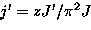(7)
Using the phase variable, the z-component of a spin is expressed as:

As shown in eq.57, the Néel state [] and the singlet state [] are both expressed equally in this `phase Hamiltonian' (eq.54). It has been shown that this formalism gives the same result as Cross and Fisher's approach, but more easily and intuitively [125,126]. Using the phase Hamiltonian approach, the effects of inter-chain interactions (J') and external magnetic field (H) have been investigated [17,18]. The results are summarized as follows:
(1)
In zero-field, the ground state is either the Néel state or spin-Peierls state, depending on the magnitude of the inter-chain interaction. The phase boundaryis first order, whereis a normalized inter-chain interaction,is a dimensionless spin-lattice coupling parameter and.
(2)
The ground state in finite magnetic field was obtained, as shown in Fig.50. The spin-Peierls phase (SP) makes a first order transition to a magnetic phase (M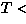) at a critical field (ht1). There is a spin-flop phase (SF) in the large j' region, but experimentally the SP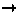SF transition may be difficult to observe, because of the narrow parameter range (B on the-axis) as the condition.Next: 6.1.2 Organic spin Peierls Up: 6.1 Introduction Previous: 6.1 Introduction